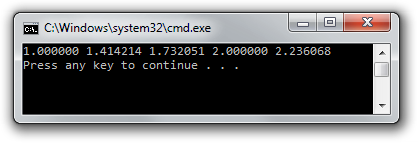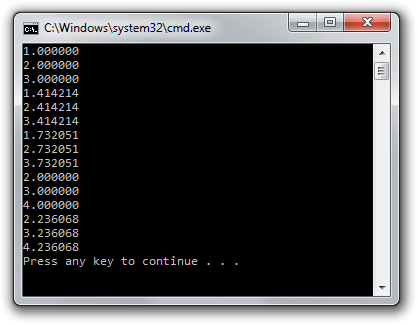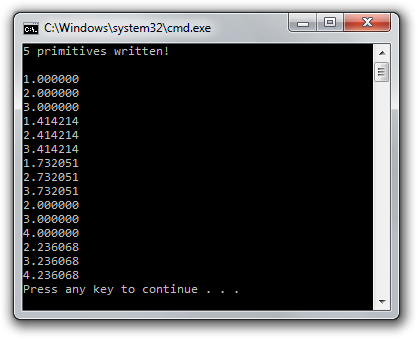# Transform feedback

Up until now we've always sent vertex data to the graphics processor and only produced drawn pixels in framebuffers in return. What if we want to retrieve the vertices after they've passed through the vertex or geometry shaders? In this chapter we'll look at a way to do this, known as transform feedback.

So far, we've used VBOs (Vertex Buffer Objects) to store vertices to be used for drawing operations. The transform feedback extension allows shaders to write vertices back to these as well. You could for example build a vertex shader that simulates gravity and writes updated vertex positions back to the buffer. This way you don't have to transfer this data back and forth from graphics memory to main memory. On top of that, you get to benefit from the vast parallel processing power of today's GPUs.

## Basic feedback

We'll start from scratch so that the final program will clearly demonstrate how simple transform feedback is. Unfortunately there's no preview this time, because we're not going to draw anything in this chapter! Although this feature can be used to simplify effects like particle simulation, explaining these is a bit beyond the scope of these articles. After you've understood the basics of transform feedback, you'll be able to find and understand plenty of articles around the web on these topics.

const GLchar* vertexShaderSrc = R"glsl(
in float inValue;
out float outValue;

void main()
{
outValue = sqrt(inValue);
}
)glsl";


This vertex shader does not appear to make much sense. It doesn't set a gl_Position and it only takes a single arbitrary float as input. Luckily, we can use transform feedback to capture the result, as we'll see momentarily.

GLuint shader = glCreateShader(GL_VERTEX_SHADER);

GLuint program = glCreateProgram();


Compile the shader, create a program and attach the shader, but don't call glLinkProgram yet! Before linking the program, we have to tell OpenGL which output attributes we want to capture into a buffer.

const GLchar* feedbackVaryings[] = { "outValue" };
glTransformFeedbackVaryings(program, 1, feedbackVaryings, GL_INTERLEAVED_ATTRIBS);


The first parameter is self-explanatory, the second and third parameter specify the length of the output names array and the array itself, and the final parameter specifies how the data should be written.

The following two formats are available:

• GL_INTERLEAVED_ATTRIBS: Write all attributes to a single buffer object.
• GL_SEPARATE_ATTRIBS: Writes attributes to multiple buffer objects or at different offsets into a buffer.

Sometimes it is useful to have separate buffers for each attribute, but lets keep it simple for this demo. Now that you've specified the output variables, you can link and activate the program. That is because the linking process depends on knowledge about the outputs.

glLinkProgram(program);
glUseProgram(program);


After that, create and bind the VAO:

GLuint vao;
glGenVertexArrays(1, &vao);
glBindVertexArray(vao);


Now, create a buffer with some input data for the vertex shader:

GLfloat data[] = { 1.0f, 2.0f, 3.0f, 4.0f, 5.0f };

GLuint vbo;
glGenBuffers(1, &vbo);
glBindBuffer(GL_ARRAY_BUFFER, vbo);
glBufferData(GL_ARRAY_BUFFER, sizeof(data), data, GL_STATIC_DRAW);


The numbers in data are the numbers we want the shader to calculate the square root of and transform feedback will help us get the results back.

With regards to vertex pointers, you know the drill by now:

GLint inputAttrib = glGetAttribLocation(program, "inValue");
glEnableVertexAttribArray(inputAttrib);
glVertexAttribPointer(inputAttrib, 1, GL_FLOAT, GL_FALSE, 0, 0);


Transform feedback will return the values of outValue, but first we'll need to create a VBO to hold these, just like the input vertices:

GLuint tbo;
glGenBuffers(1, &tbo);
glBindBuffer(GL_ARRAY_BUFFER, tbo);


Notice that we now pass a nullptr to create a buffer big enough to hold all of the resulting floats, but without specifying any initial data. The appropriate usage type is now GL_STATIC_READ, which indicates that we intend OpenGL to write to this buffer and our application to read from it. (See reference for usage types)

We've now made all preparations for the rendering computation process. As we don't intend to draw anything, the rasterizer should be disabled:

glEnable(GL_RASTERIZER_DISCARD);


To actually bind the buffer we've created above as transform feedback buffer, we have to use a new function called glBindBufferBase.

glBindBufferBase(GL_TRANSFORM_FEEDBACK_BUFFER, 0, tbo);


The first parameter is currently required to be GL_TRANSFORM_FEEDBACK_BUFFER to allow for future extensions. The second parameter is the index of the output variable, which is simply 0 because we only have one. The final parameter specifies the buffer object to bind.

Before doing the draw call, you have to enter transform feedback mode:

glBeginTransformFeedback(GL_POINTS);


It certainly brings back memories of the old glBegin days! Just like the geometry shader in the last chapter, the possible values for the primitive mode are a bit more limited.

• GL_POINTSGL_POINTS
• GL_LINESGL_LINES, GL_LINE_LOOP, GL_LINE_STRIP, GL_LINES_ADJACENCY, GL_LINE_STRIP_ADJACENCY
• GL_TRIANGLESGL_TRIANGLES, GL_TRIANGLE_STRIP, GL_TRIANGLE_FAN, GL_TRIANGLES_ADJACENCY, GL_TRIANGLE_STRIP_ADJACENCY

If you only have a vertex shader, as we do now, the primitive must match the one being drawn:

glDrawArrays(GL_POINTS, 0, 5);


Even though we're now working with data, the single numbers can still be seen as separate "points", so we use that primitive mode.

End the transform feedback mode:

glEndTransformFeedback();


Normally, at the end of a drawing operation, we'd swap the buffers to present the result on the screen. We still want to make sure the rendering operation has finished before trying to access the results, so we flush OpenGL's command buffer:

glFlush();


Getting the results back is now as easy as copying the buffer data back to an array:

GLfloat feedback;
glGetBufferSubData(GL_TRANSFORM_FEEDBACK_BUFFER, 0, sizeof(feedback), feedback);


If you now print the values in the array, you should see the square roots of the input in your terminal:

printf("%f %f %f %f %f\n", feedback, feedback, feedback, feedback, feedback);Congratulations, you now know how to make your GPU perform general purpose tasks with vertex shaders! Of course a real GPGPU framework like OpenCL is generally better at this, but the advantage of transform feedback is that you can directly repurpose the data in drawing operations, by for example binding the transform feedback buffer as array buffer and performing normal drawing calls.

If you have a graphics card and driver that supports it, you could also use compute shaders in OpenGL 4.3 instead, which were actually designed for tasks that are less related to drawing.

You can find the full code here.

## Feedback transform and geometry shaders

When you include a geometry shader, the transform feedback operation will capture the outputs of the geometry shader instead of the vertex shader. For example:

// Vertex shader
in float inValue;
out float geoValue;

void main()
{
geoValue = sqrt(inValue);
}
)glsl";

layout(points) in;
layout(triangle_strip, max_vertices = 3) out;

in float[] geoValue;
out float outValue;

void main()
{
for (int i = 0; i < 3; i++) {
outValue = geoValue + i;
EmitVertex();
}

EndPrimitive();
}
)glsl";


The geometry shader takes a point processed by the vertex shader and generates 2 more to form a triangle with each point having a 1 higher value.

GLuint geoShader = glCreateShader(GL_GEOMETRY_SHADER);

...



Compile and attach the geometry shader to the program to start using it.

const GLchar* feedbackVaryings[] = { "outValue" };
glTransformFeedbackVaryings(program, 1, feedbackVaryings, GL_INTERLEAVED_ATTRIBS);


Although the output is now coming from the geometry shader, we've not changed the name, so this code remains unchanged.

Because each input vertex will generate 3 vertices as output, the transform feedback buffer now needs to be 3 times as big as the input buffer:

glBufferData(GL_ARRAY_BUFFER, sizeof(data) * 3, nullptr, GL_STATIC_READ);


When using a geometry shader, the primitive specified to glBeginTransformFeedback must match the output type of the geometry shader:

glBeginTransformFeedback(GL_TRIANGLES);


Retrieving the output still works the same:

// Fetch and print results
GLfloat feedback;
glGetBufferSubData(GL_TRANSFORM_FEEDBACK_BUFFER, 0, sizeof(feedback), feedback);

for (int i = 0; i < 15; i++) {
printf("%f\n", feedback[i]);
}Although you have to pay attention to the feedback primitive type and the size of your buffers, adding a geometry shader to the equation doesn't change much other than the shader responsible for output.

The full code can be found here.

## Variable feedback

As we've seen in the previous chapter, geometry shaders have the unique property to generate a variable amount of data. Luckily, there are ways to keep track of how many primitives were written by using query objects.

Just like all the other objects in OpenGL, you'll have to create one first:

GLuint query;
glGenQueries(1, &query);


Then, right before calling glBeginTransformFeedback, you have to tell OpenGL to keep track of the number of primitives written:

glBeginQuery(GL_TRANSFORM_FEEDBACK_PRIMITIVES_WRITTEN, query);


After glEndTransformFeedback, you can stop "recording":

glEndQuery(GL_TRANSFORM_FEEDBACK_PRIMITIVES_WRITTEN);


Retrieving the result is done as follows:

GLuint primitives;
glGetQueryObjectuiv(query, GL_QUERY_RESULT, &primitives);


You can then print that value along with the other data:

printf("%u primitives written!\n\n", primitives);Notice that it returns the number of primitives, not the number of vertices. Since we have 15 vertices, with each triangle having 3, we have 5 primitives.

Query objects can also be used to record things such as GL_PRIMITIVES_GENERATED when dealing with just geometry shaders and GL_TIME_ELAPSED to measure time spent on the server (graphics card) doing work.

See the full code if you got stuck somewhere on the way.

## Conclusion

You now know enough about geometry shaders and transform feedback to make your graphics card do some very interesting work besides just drawing! You can even combine transform feedback and rasterization to update vertices and draw them at the same time!

## Exercises

• Try writing a vertex shader that simulates gravity to make points hover around the mouse cursor using transform feedback to update the vertices. (Solution)